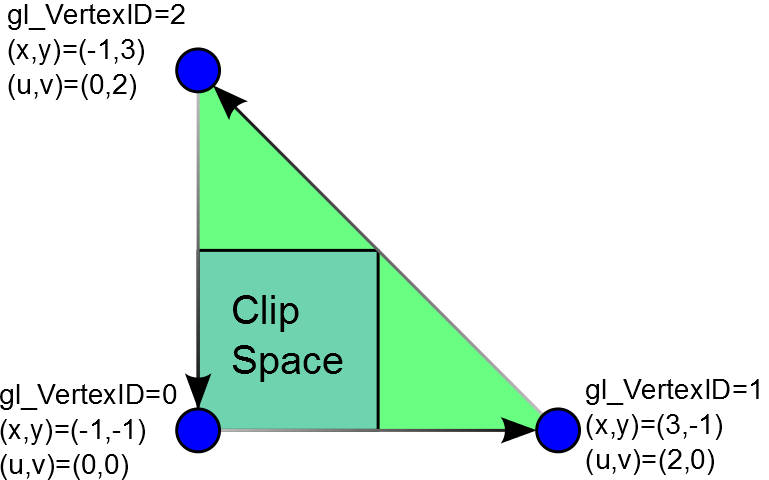# Rendering a Screen Covering Triangle in OpenGL (with no buffers)

GLSL
OpenGL
Author

Randall Rauwendaal

Published

June 14, 2014

This one has been on the backlog for ages now.  Anyway, this is an OpenGL adaptation of a clever trick that's been around for quite awhile and described in DirectX terms by Cort Stratton (@postgoodism) in the "An interesting vertex shader trick" on #AltDevBlogADay.

It describes a method for rendering a triangle that covers the screen with no buffer inputs.  All vertex and texture coordinate information are generated solely from the vertexID.  Unfortunately, because OpenGL uses a right-handed coordinate system while DirectX uses a left-handed coordinate system the same vertexID transformation used for DirectX won't work in OpenGL.  Basically, we need to reverse the order of the triangle vertices so that they are traversed counter-clockwise as opposed to clockwise in the original implementation. So, after a bit of experimentation I came up with the following adaptation for OpenGL:

``````void main()
{
float x = -1.0 + float((gl_VertexID & 1) << 2);
float y = -1.0 + float((gl_VertexID & 2) << 1);
gl_Position = vec4(x, y, 0, 1);
}``````

This transforms the `gl_VertexID` as follows:

`gl_VertexID=0 -> (-1,-1)` `gl_VertexID=1 -> ( 3,-1)` `gl_VertexID=2 -> (-1, 3)`

We can easily add texture coordinates to this as well:

out vec2 texCoord;

``````void main()
{
float x = -1.0 + float((gl_VertexID & 1) << 2);
float y = -1.0 + float((gl_VertexID & 2) << 1);
texCoord.x = (x+1.0)*0.5;
texCoord.y = (y+1.0)*0.5;
gl_Position = vec4(x, y, 0, 1);
}``````

Which is going to provide in that homogeneous clip space region a position value varying from -1 to 1 and texture coordinates varying from 0 to 1 exactly as OpenGL would expect, all without need to any create any buffers. All you have to do is make single call to `glDrawArrays` and tell it to render 3 vertices:

``glDrawArrays( GL_TRIANGLES, 0, 3 );``

This draw a triangle that looks like the following:It's surprising how often this comes in handy, in a later post I'll describe how to adapt this trick to efficiently access the elements of a 3D texture.  It also amuses me greatly that Iñigo Quilez's amazing demo/presentation "Rendering World's With Two Triangles" could actually be renamed "Renderings Worlds With One Triangle."

## Citation

BibTeX citation:
``````@online{rauwendaal2014,
author = {Randall Rauwendaal},
title = {Rendering a {Screen} {Covering} {Triangle} in {OpenGL} (with
No~Buffers)},
date = {2014-06-14},
url = {https://raegnar.github.io/rauwendaal.net//posts/2014_06_14 - Rendering a Screen Covering Triangle in OpenGL (with no buffers)},
langid = {en}
}
``````